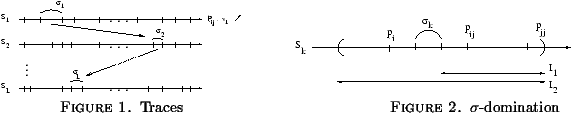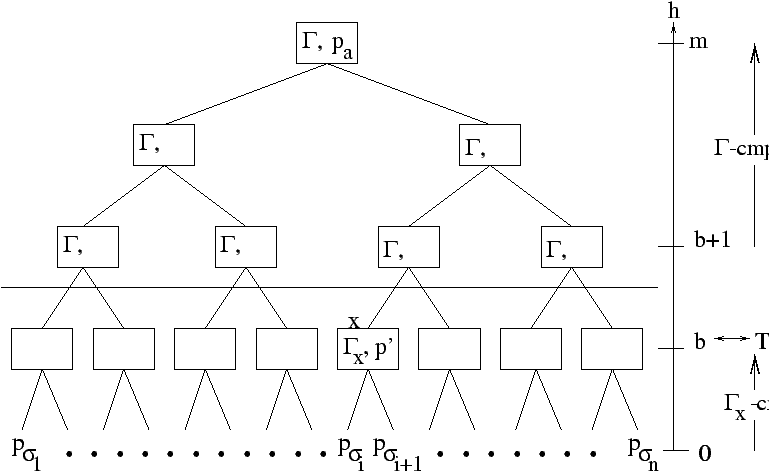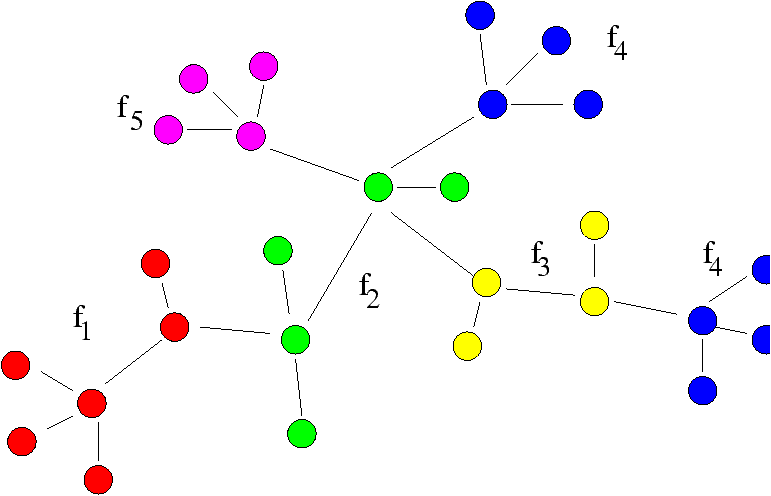Nearest-Neighbour Search in High Dimension and Molecular Clustering

Frédéric Cazals

Algorithms project, INRIA Rocquencourt

Algorithms Seminar

June 30, 1997

[summary by Frédéric Cazals]

A properly typeset version of this document is available in postscript and in pdf.

If some fonts do not look right on your screen, this might be fixed by configuring your browser (see the documentation here).

## 1   Introduction and prerequisites

### 1.1   Problem statement

Given a set of points P={p1,...,pn} Ì Rd, the nearest-neighbour (NN) and k nearest neighbours (k-NN) problems can be stated as follows: pre-process P in order to return as fast as possible the nearest or k nearest neighbour(s) of an arbitrary point q according to any Euclidian metric d(p,q) = (åi=1d (pi - qi)2)1/2. A weakened version of the NN problem consists in returning a point p' which e-approximates the NN p of q in the sense d(p',q) d(p,q) £ 1+e for any e > 0. If one denotes pi1,...,pin the points of P sorted by increasing distance to q, an equivalent formulation for the k-NN problem consists in returning a subset S={s1,...,sk} with d(q,sj) £ (1+e)d(q,pij) for j=1,...,k.

The naive algorithm to compute the NN of a point q consists in checking all the points of P and returning the closest, which has complexity O(dn). On the other hand, the most sophisticated algorithms known until recently had complexities in O(exp(d) log n) with exp(d) a function growing at least as quickly as 2d---see e.g., . So that whenever d ³ log n nothing better than the brute force method was known!

Kleinberg's break-through  has been to get around the exponential difficulty by an heavy use of random sampling aiming at ``comparing'' the points of P through their projections on random lines passing through the origin rather than decomposing the d-dimensional space containing them. The first result is an algorithm returning an approximation of the k-NN in a deterministic way but with an exponential time/space pre-processing. The second algorithm returns an approximation of the NN in a randomised way but with a polynomial pre-processing only. This talk presents these two algorithms and discusses their potential use to a clustering problem arising in chemistry---see section 4.

### 1.2   Prerequisites

#### 1.2.1   A geometric lemma

The core idea of Kleinberg's method lies in the following property:
Lemma 1   Let x and y be two vectors of Rd such that ||y||/||x|| ³ 1+g with g £ 1/2. Then, if v is a random vector on the unit sphere Sd-1 we have Pr[|x · v| ³ |y · v|] £ 1/2 - g/3.
Intuitively, short vectors ``should not defeat too often'' longer ones when comparing their projection on a random line determined by a vector on Sd-1. In order to compare two vectors from their projections, the key point is therefore to use a large enough set of lines to capture the probabilistic property contained in the above theorem.

#### 1.2.2   Empirical measures and Vapnik-Chervonenkis bounds

Let (W, F,µ) be a probability space, S Ì F a set of events, and X1,X2,...,Xn n random variables following the law µ. If one calls the empirical measure of an event s the fraction of Xi's falling into s, the quantity
DSn =
 sup s Î S
½
½
½
½
1s(X1)+...+1s(Xn)
n
-µ(s) ½
½
½
½
measures the maximum difference over the class S between the empirical measure and the probability. It is a random variable and Vapnik-Chervonenkis's contribution  has been to elucidate the conditions under which it converges in probability to zero, that is the conditions under which limn ® ¥ Pr[DSn > e] = 0 for any e. To sketch this contribution, let a range-space be a couple ( P, R)= ((W, F,µ), R Ì F ). We shall say that a set A of finite cardilality is shattered by R if " a Î 2A  \$ rÎ R such that a=r Ç A. The dimension of Vapnik-Chervonenkis of ( P, R) is the cardinality of the biggest A Ì W shattered by R.
Definition 1   A g-sample for ( P, R) is a finite set A Ì W such that |µ(r)-| r È A |/| A || £ g, " rÎ R.
Theorem 1  []   For a range space of dimension k, a random sample of size
l ³
16
g2
(k log
16k
g2
+ log
4
d
)
is a g-sample with a probability at least 1-d.

#### 1.2.3   Exceptional and r-distinguishing sets

As pointed out above, we are interested in comparing points with respect to their projections on vectors from Sd-1. For two vectors x and y with ||y|| / ||x|| ³ 1+g we call their exceptional set
W
 x,y
= { v Î Sd-1 such that | x · v| ³ | y · v | }.
And a random set of vectors V from Sd-1 is called r-distinguishing if
" W
 x,y
,  µ(W
 x,y
)<r Þ | V Ç W
 x,y
| / | V | < 1/2.
More prosaically, a set V is r-distinguishing if a majority of its points do not fall into some exceptional set of size smaller than r.

#### 1.2.4   Hyper-planes arrangements

An arrangement of n hyper-planes in Rd is said to be in general position if any d hyper-planes have a unique point in common, and any d+1 hyper-planes do not share a point. Given a hyper-plane h and a point p, p is either above, on, or below h, which is called its position. A face on an arrangement is the set of points having the same position with respect to all the hyper-planes. The dimension of a face is its affine dimension. It is known from  that
Theorem 2   The number of d-faces of an arrangement of n hyper-planes in general position is fd(n)=åi=0d (
 n i
).

#### 1.2.5   Digraphs

A complete digraph G on n vertices 1,2,...,n is a directed graph which contains for any pair of vertices {i,j} either the edge (i,j) or (i,j). An apex of G is a vertex with a directed path of length at most two to any vertex. At least, an apex ordering of G is an ordering i1,...,in of its vertices such that ik is an apex for the sub-digraph G[ik,ik+1,...,in]. The following is straightforward:
Theorem 3   Every n-node complete digraph has an apex ordering computable in O(n2).

### 1.3   First results

The following theorems can be proved:
Lemma 2   Let µ be the uniform measure on Sd-1. The dimension of the range-space
((Sd-1, F , µ), { W
 x,y
|µ(W
 x,y
) £ r })
is less than d' = 8(d+1)log (4d+4).

Lemma 3   There exists c0 such that a random sample of Sd-1 of size f(d,g) is a g/2-sample for the range-space ((Sd-1, F, µ), { Wx,y|µ(Wx,y) £ r }) with f(d,g)= c0/g2 (d' log d'/g2 + log 1/d)= q(d log2 d).

Corollary 1   A set V of f(g,d) vectors from Sd-1 is (1/2-g)-distinguishing with a probability at least 1-d.

## 2   First algorithm

### 2.1   Construction of the data structure

This algorithm returns an approximation of the k-NN of a point q. To build the data structure from which it does so, we first draw uniformly at random a set V of L = f(e/3,d) = q(d log2 d) vectors from Sd-1. Then for each vector vl Î V, the following is done: 1. compute vl · pij with pij=(pi+pj)/2, 1£ i,j£ n; 2. sort the pij according to the values of vl · pij and denote Sl the list obtained. The list of lists S1,...,SL is denoted S.

For each such list, a pair of consecutive entries is called a primitive interval, and a sequence of primitive intervals is called a trace---see Figure 1. The maximum number of traces is upper-bounded by (n2)L = nO(d log2 d). But a trace is realizable if
\$ q Î Rd, " k=1,...,L, vk · p
 (k) i1i2
< vk · q < vk · p
 (k) i3i4
.
So that realizable traces are defined with respect to the Ln2 hyper-planes vk · (pi1i2(k) - x). And from theorem 2, the number of such traces is åi=0d (
 Ln2 i
) = O(n log d)2d.

Definition 2   For a realizable trace s=s1 ··· sk ··· sL, pi is said to s-dominate pj in Sk if pij lies in the interval (sk,...,pjj).

For each realizable trace s, the construction is as follows: (1.) build a complete digraph Gs on {1,2,...,n} with the edge (i,j) if pi s-dominates pj in half of the lists of S; (2.) build an apex ordering (s,Gs) of the nodes of Gs.

The idea behind the domination definition is depicted on Figure 2: if pij falls in the desired interval denoted I1, then pi Î I2 and we have |vk · (pi-q)| < |vk · (pj-q)|.### 2.2   Algorithm

To process a query associated to a point q: 1. compute s(q)=s1(q) ··· sL(q) with sk(q) the primitive interval from Sl containing vl · q; 2. retrieve the apex ordering associated to s(q) and return the k first entries.

This algorithm therefore returns an e-approximation of the k nearest neighbours of a point q in a deterministic fashion, so that the answer is guaranteed to be correct if the random sample V is actually (1/2-e/3)-distinguishing---see §1.2.3 and Corollary 1. Roughly speaking, the correctness of the algorithm comes from the fact that if a non-desired point p1 has been returned instead of a desired point p2, then p1 dominated p2 in more than half of the lists of S, and the sample V was not distinguishing enough with respect to the exceptional set Wq-p1,q-p2.

The pre-processing requires O (Ln2 (n log d)(2d) ) time and O (n (n log d)(2d) ) space. The cost of a query is O(k+L (d+log n))=O(k + (d log2 d) (d + log n)).

## 3   Second algorithm

As opposed to the first algorithm, the second one does not try to compute a partition of the requests' space and proceeds in two steps. The first one consists in drawing a random sample from P of the appropriate size and returning the closest point to q. The second one compares iteratively q to pairs of points of P in a tournament way depicted on Figure 3. Assuming that n=2m, the overview of this second stage is the following:
1. a random sample V of Q(d log2 n (log2 d + log d log log n)) vectors is drawn uniformly on Sd-1, and a multi-set Gv of V is assigned to each internal node v of the binary tree whose leaves are a permutation of the points of P. For a query point q, two points pi and pj of P, and a multi-set Gv, pi is said to dominate pj if |vk · (pi-q)| < |vk · (pj-q)| holds for a majority of vectors vk in Gv. Otherwise pj dominates pi;
2. each internal node v of the tree is assigned its dominating child for the multi-set Gv.
The point eventually returned is the best candidate from the two points returned by the two steps. It can be shown that an e-approximation is returned with a probability greater than 1-d in O(n + d log3 n) time with a space requirement of n| V |.Figure 1: Tournament treeFigure 2: Molecule and fragments## 4   Application to molecular clustering

Suppose we are given a set of d molecular fragments, say F={f1,...,fd} and a set M={m1,...,mNm} of Nm molecules, each described by a set of fragments of F---see Figure 4. We shall represent a molecule mi by a sequence of d boolean values mi=bi, 1 bi, 2 ··· bi,d with bi, j=1 if mi contains fj and bi, j=0 otherwise. This formulation fails to report multiple occurrences of a given fragment in a molecule, but it has the nice property that a molecule is represented by a point on the hyper-cube Hd={0,1}d. Given two molecules, we call their similarity the number of common fragments that is the quantity sim(mi,mj) = åk=1d bi, k· bj, k.

Given the set M we are interested in the following problem: find a partition of M into subsets of neighbours or clusters. To do so, one way to proceed see  consists in first building a Minimum Spanning Tree on the input data set, second removing those ``too long'' edges from the MST, and third computing the connected components we are left with. The key point lies in the MST computation, and it is shown in  that
Theorem 4   A MST on n points in dimension d can be found in
O(2dn2-1/2d+1 (log n)1-1/2d+1) time.

Unfortunately for our concern where d can range from 500 to 2000, the 2d constant is prohibitive. Kleinberg's algorithms customised to the hyper-cube setting could make Yao's algorithm interesting in practice.

## References


Arya (S.), Mount (D. M.), Netanyahu (N. S.), Silverman (R.), and Wu (A. Y.). -- An optimal algorithm for approximate nearest neighbour searching. In Proceedings of the Fifth Annual ACM-SIAM Symposium on Discrete Algorithms. pp. 573--582. -- New York, 1994.


Edelsbrunner (Herbert). -- Algorithms in combinatorial geometry. -- Springer-Verlag, Berlin, 1987, EATCS Monographs on Theoretical Computer Science, vol. 10, xvi+423p.


Jain (Anil K.) and Dubes (Richard C.). -- Algorithms for clustering data. -- Prentice-Hall Inc., Englewood Cliffs, NJ, 1988, Prentice-Hall Advanced Reference Series, xiv+320p.


Kleinberg (J.). -- Two algorithms for nearest-neighbour search in high dimension. In ACM STOC. -- El Paso, Texas, USA, 1997.


Vapnik (N.) and Chervonenkis (A.). -- On the uniform convergence of relative frequencies of events to their probabilities. Theory of Probability and its Applications, vol. 16, n°2, 1971, pp. 264--280.


Yao (Andrew Chi Chih). -- On constructing minimum spanning trees in k-dimensional spaces and related problems. SIAM Journal on Computing, vol. 11, n°4, 1982, pp. 721--736.

This document was translated from LATEX by HEVEA.